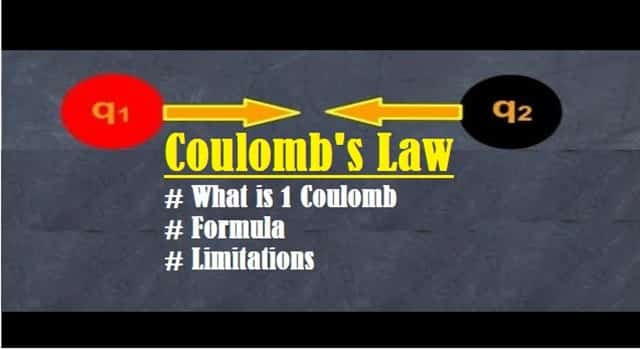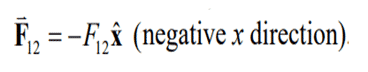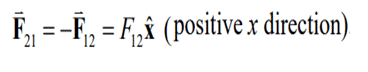Sunday, September 19, 2021
HomeCoulomb’s LawCoulomb's Law Equation, Principle, Coulomb's Constant, Inverse Square Law

# Coulomb’s Law Equation, Principle, Coulomb’s Constant, Inverse Square Law

Coulomb’s Law is a vector equation that determines the electrostatic force between the two charges. This post will discuss What is Coulomb’s Law, its principle, properties of electric charge, Coulomb’s Constant, Equation, Inverse Square Law, advantages and limitations.

## What is Coulomb’s Law

Coulomb’s Law states that – “The electrostatic force (attraction or repulsion) between two electrically charged bodies is directly proportional to the product of charge and inversely proportional to the square of the distance between the center of the bodies which are charged”. Due to this inverse square relation, the law is also called Coulomb’s Inverse Square Law.Fig. 1 – Introduction to Coulomb’s Law | Inverse Square Law

Static Electricity was invented by a Greek Philosopher Thales of Miletus in 600BC. At that time, all he observed was that if two bodies charged through static electricity are brought towards each other, they would either attract (due to opposite charges) or repel (due to exact charges).

In 1785, Charles Augustin de Coulomb, a French physicist, was able to conclude with the mathematical relationship between the charged objects through the law that is known after his name as Coulomb’s law.Fig. 2 – Image of Augustin de Coulomb

## Properties of Electric Charge

Before we understand the principle of Coulomb’s Law, it is important to know the properties of electric charge. They are:

• The law of conservation of energy states that energy can neither be created nor destroyed. It can only change from one form to another.
• The unit of charge is coulomb and is a scalar quantity.
• There is a free movement of charge through the conductors and no movement at all in insulators.
• Point of charge is when the object size is smaller than the distance between the charges.
• It is possible to add the charges which have magnitude but no direction.

## What is 1 Coulomb of Charge

1 Coulomb is a charge that tends to repel a charge with an equal magnitude of the same sign. That is, the charge on both objects should be identical. Also, the factor that needs to be considered here is that the value of the Coulomb force stays true only if the charges are static.

It can also be defined as the quantity of charge, when placed at a distance of 1 meter in air or vacuum from an equal and similar charge, experiences a repulsive force of 9 x 109 N.

## Coulomb’s Law Equation | Coulomb’s Constant

Coulombs Law Equation is defined as:

F = Kq1 q2d2

Where,

• K is Coulomb’s Constant, k≈ 9 × 109 N m2/c2
• q1 is the charge of the first body
• q2 is the charge of the second body
• d is the distance between the two bodies
• F is the magnitude of force between the two bodies

Coulomb’s Constant (k) is also referred as Electrostatic Constant. The body’s charge is in Coulomb; the distance is in meters, and the force is calculated in Newton. Based on Newton’s third law, this is the magnitude of force which each charge exerts on the other charge.

The force is mutually repulsive if the charges q1 and q2 are of the same sign (both positive or both negative) and the force is said to be mutually attractive if the charges are of opposite signs (one positive, one negative). This is illustrated in the Fig.3.Fig. 3 – Illustration of Coulomb’s Law Equation (a) Repulsive Force (b) Attractive Force

## Principle of Coulomb’s Law

The principle of Coulombs Law can best be understood with the help of an example. Let us consider two particles A and B. Charge on particle A is q1 and B has charge q2, separated by a distance x. Particles A and B both are negatively charged. Let us assume the values to be:F12 denotes the force acting on A with charge q1 due to the presence of object B with charge q2. This is illustrated in the Fig. 4 (a).  As both the particles are negatively charged, the force is repulsive and is given by the equation:Newtons third law states that for every action, there is an equal and opposite reaction and the force on particle B from particle A is given by the equation:By using Coulomb’s Law, Magnitude of Force is devised.Similarly, if we consider three charges [shown in Fig. 4 (b)] with equal negative charge then the Force will be repulsive. Though the magnitude of all the forces are equal, the direction of Forces are not always the same.

(a) Two Charges (b) Three Charges)

Here are significant advantages of Coulomb’s Law:

• It helps to find the distance between the two electrically charged objects.
• The direction between the two charged objects can also be found out using the mathematical expression of Coulomb’s law.
• The Vector field of the objects can also be calculated using the formula.
• Electric field formula can also be derived easily from the law.

## Limitation of Coulomb’s Law

Here are few limitations of Coulomb’s Law:

• Coulomb’s law is only accurate when the average number of molecules in the objects is not extensive. Hence, the law cannot be used for big things like planets.
• The law only applies if the objects are at rest. For moving objects, it is not applicable.
• It is difficult to calculate the value of d, which is the distance between them if the shapes of the objects are arbitrary.
• The law can only be used where the rule of inverse proportion is obeyed.
```Also Read:
How Capacitor works(Opens in a new browser tab)
Power Factor - Power Triangle, Types, PFC, Applications, Advantages
Electric Field - Gauss's & Coulomb's Law, Electric Field Lines, Applications
```Chakrasthitha
Chakrasthitha is a B.E (Medical Electronics) and has work experience in MatLab and Lab View Software as Design Engineer at BCS innovations and Manipal hospital as Biomedical Engineer. She is an author, editor and partner at Electricalfundablog.
RELATED ARTICLES